### Home > CALC > Chapter 6 > Lesson 6.1.2 > Problem6-26

6-26.
1. Examine the following derivatives. Consider the multiple tools available for finding derivatives and use the best strategy. After finding each derivative, write a short description of your method. Homework Help ✎

1.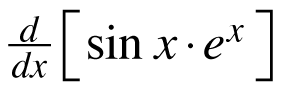2.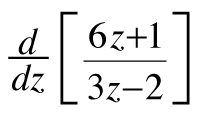3.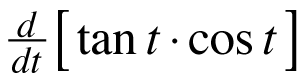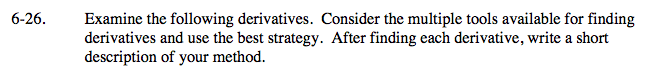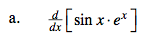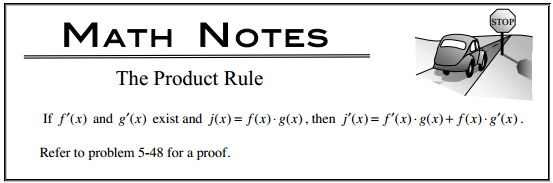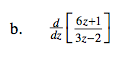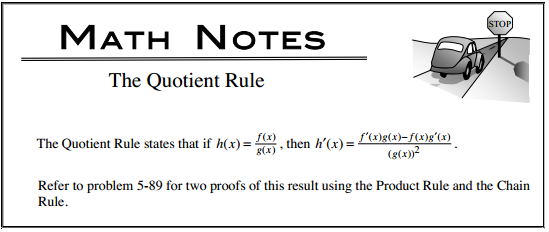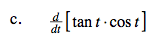$\text{tan}(t)=\frac{\text{sin}(t)}{\text{cos}(t)}$